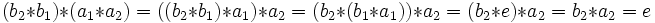# Left-invertible elements of monoid form submonoid

## Statement

Let$M$ be a monoid with neutral element$e$. Then, the set of left-invertible elements of$M$ form a submonoid of$M$.

## Related facts

Inverse map is involutive: Specifically the reversal law for inverses.

## Proof

Given: A monoid$M$ with neutral element$e$

To prove:$e$ is left-invertible, and further, if$a_1, a_2$ are left-invertible elements of$M$, so is$a_1 * a_2$

Proof: Clearly,$e$ is left-invertible, since$e * e = e$.

Suppose$a_1,a_2$ are left-invertible and$b_1,b_2$ are left inverses for$a_1, a_2$. Then consider:$(b_2 * b_1) * (a_1 * a_2) = ((b_2 * b_1) * a_1) * a_2 = (b_2 * (b_1 * a_1)) * a_2 = (b_2 * e) * a_2 = b_2 * a_2 = e$

Thus,$b_2 * b_1$ is a left inverse for$a_1 * a_2$, so$a_1 * a_2$ is left-invertible, completing the proof.